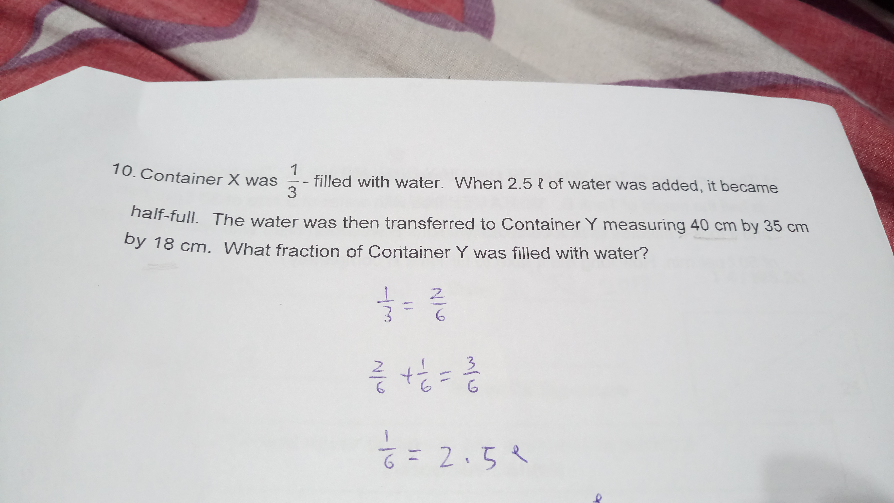# QuestionYour kid was on the right track….

½ – 1/3 = 1/6

1/6 = 2.5 l = 2500 cm3

½ = 2500 × 3 = 7500 cm (transferred to Y)

Total volume of Y -> 40 × 35 × 18 = 25200 cm3

Fraction -> 7500/25200 = 25/84

0 Replies 1 Like ✔Accepted Answer